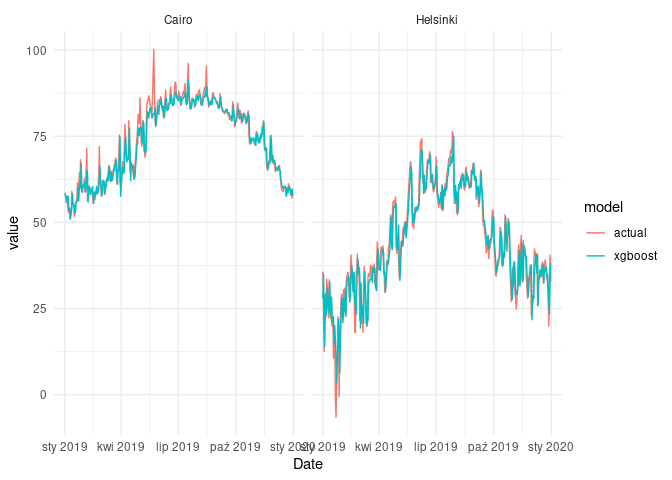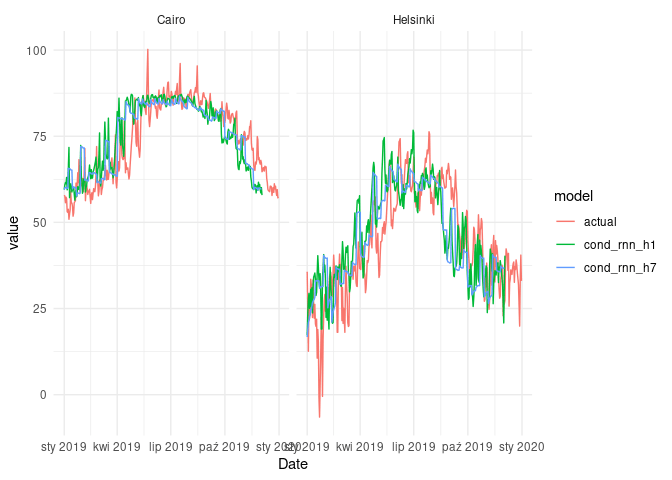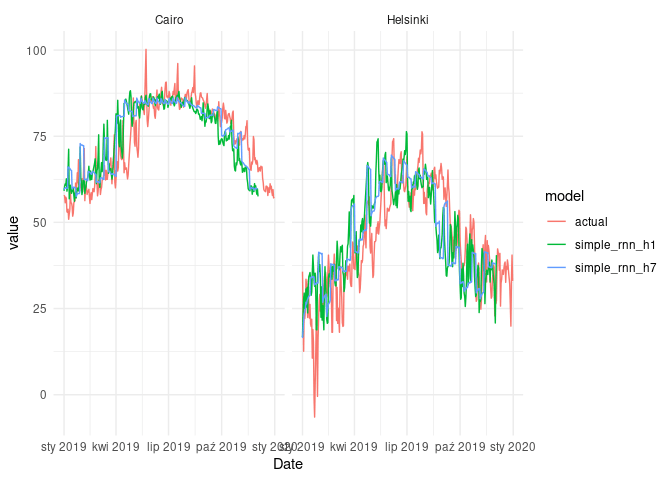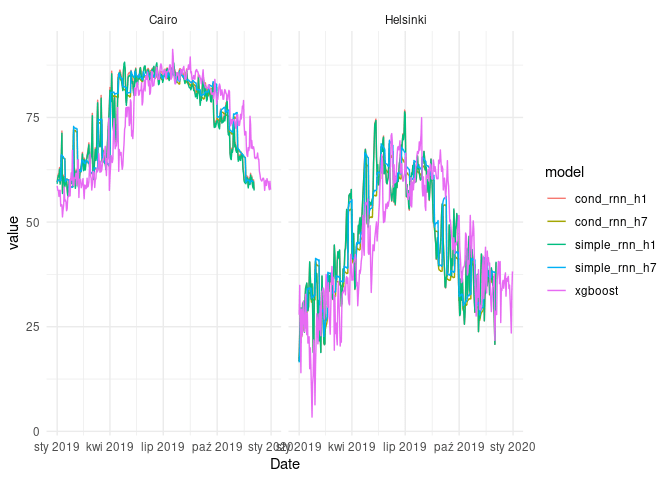[This article was first published on R on krzjoa, and kindly contributed to R-bloggers]. (You can report issue about the content on this page here)
Want to share your content on R-bloggers? click here if you have a blog, or here if you don't.Conditional RNN is one of the possible solutions if we’d like to make use of static features in time series forecasting. For example, we want to build a model, which can handle multiple time series with many different characteristics. It can be a model for demand forecasting for multiple products or a unified model forecasting temperature in places from different climate zones.

We have at least a couple of options to do so. They are described in detail in the following thread on StackOverlow. According to the answers, the best way to add static features is to use this values to produce an initial hidden state of the recurrent layer. The proposed solution was implemented as a Keras wrapper to recurrent layers (in Python).

This post is a trial to implement conditional RNN in keras for R.

We’ll use a piece of data from an experiment performed by the author of the aforementioned Keras wrapper. We’re selecting two cities with extreme temperatures, e.g. Cairo and Helsinki.

```library(data.table, warn.conflicts = FALSE)
library(dplyr, warn.conflicts = FALSE)
library(lubridate)
library(ggplot2)
library(imputeTS
library(timetk)
library(rsample)
library(parsnip)

# url <- "https://github.com/philipperemy/cond_rnn/raw/master/examples/temperature/city_temperature.csv.zip"
file_path <- "city_temperature.csv.zip"
csv_path <- "city_temperature.csv"

# unzip(file_path)

setDT(city_temperature)

selected_cities <-
city_temperature[City %chin% c('Cairo', 'Helsinki')]

selected_cities[
, Date := ymd(glue::glue("{Year}-{Month}-{Day}", .envir = .SD))
]

selected_cities <-
selected_cities[, .(City, Date, AvgTemperature)]
setorder(selected_cities, City, Date)

plt <-
ggplot(selected_cities) +
geom_line(aes(Date, AvgTemperature, col = City)) +
theme_minimal() +
ggtitle("Temperature: Cairo vs Helsinki")

plt
```There is a couple of outliers and we can safely assume they simply indicate lack of data. We’ll replace it with interpolated values.

Initially, I’ve chosen Oslo, but there were a few corrupted year numbers:

```city_temperature[City == 'Oslo' & Year == 200]

## Region Country State City Month Day Year AvgTemperature
## 1: Europe Norway Oslo 12 1 200 -99
## 2: Europe Norway Oslo 12 2 200 -99
## 3: Europe Norway Oslo 12 3 200 -99
## 4: Europe Norway Oslo 12 4 200 -99
## 5: Europe Norway Oslo 12 5 200 -99
## 6: Europe Norway Oslo 12 6 200 -99
## 7: Europe Norway Oslo 12 7 200 -99
## 8: Europe Norway Oslo 12 8 200 -99
## 9: Europe Norway Oslo 12 9 200 -99
## 10: Europe Norway Oslo 12 10 200 -99
## 11: Europe Norway Oslo 12 11 200 -99
## 12: Europe Norway Oslo 12 12 200 -99
## 13: Europe Norway Oslo 12 13 200 -99
## 14: Europe Norway Oslo 12 14 200 -99
## 15: Europe Norway Oslo 12 15 200 -99
## 16: Europe Norway Oslo 12 16 200 -99
## 17: Europe Norway Oslo 12 17 200 -99
## 18: Europe Norway Oslo 12 18 200 -99
## 19: Europe Norway Oslo 12 19 200 -99
## 20: Europe Norway Oslo 12 20 200 -99
## 21: Europe Norway Oslo 12 21 200 -99
## 22: Europe Norway Oslo 12 22 200 -99
## 23: Europe Norway Oslo 12 23 200 -99
## 24: Europe Norway Oslo 12 24 200 -99
## 25: Europe Norway Oslo 12 25 200 -99
## 26: Europe Norway Oslo 12 26 200 -99
## 27: Europe Norway Oslo 12 27 200 -99
## 28: Europe Norway Oslo 12 28 200 -99
## 29: Europe Norway Oslo 12 29 200 -99
## 30: Europe Norway Oslo 12 30 200 -99
## 31: Europe Norway Oslo 12 31 200 -99
## Region Country State City Month Day Year AvgTemperature

# Cleaning the data
selected_cities[AvgTemperature == -99.0, AvgTemperature := NA]
selected_cities[, AvgTemperature := na_interpolation(AvgTemperature)]

plt <-
ggplot(selected_cities) +
geom_line(aes(Date, AvgTemperature, col = City)) +
theme_minimal() +
ggtitle("Temperature: Cairo vs Helsinki")

plt
# plotly::ggplotly(plt)
``````library(tsibble, warn.conflicts = FALSE, quietly = TRUE)

duplicates(selected_cities, key = City, index = Date)

## # A tibble: 4 × 3
## City Date AvgTemperature
## <chr> <date> <dbl>
## 1 Cairo 2015-12-30 60.5
## 2 Cairo 2015-12-30 57.9
## 3 Helsinki 2015-12-30 26.6
## 4 Helsinki 2015-12-30 25.4

# Removing dupicates for
selected_cities <-
selected_cities[, .(AvgTemperature = max(AvgTemperature)) , by = .(City, Date)]

train <- selected_cities[Date < as.Date('2019-01-01')]
test <- selected_cities[
Date >= as.Date('2019-01-01') & Date <= as.Date('2019-12-31')
]
```

## Baseline model - one xgboost model for both cities

As a baseline model, we’ll train a `xgboost` model using `parsnip` API and `modeltime`. We create a `data.frame` of lagged variables to feed the model. We use 28 lags - the same value will be later used as a length of input to the recurrent neural netowrk models. We also mix the data belonging to different cities, so there are no separate models for each city.

```library(modeltime)

lagged_selected_cities <-
selected_cities %>%
group_by(City) %>%
tk_augment_lags(AvgTemperature, .lags = 1:28) %>%
ungroup()

setDT(lagged_selected_cities)

train <- lagged_selected_cities[Date < as.Date('2019-01-01')]
test <- lagged_selected_cities[
Date >= as.Date('2019-01-01') & Date <= as.Date('2019-12-31')
]

lagged_variables <- glue::glue("AvgTemperature_lag{1:28}")
formula_rhs <- paste0(lagged_variables, collapse = " + ")
model_formula <- as.formula(
glue::glue("AvgTemperature ~ {formula_rhs}")
)

model_xgboost <-
boost_tree(mode = "regression") %>%
set_engine("xgboost") %>%
fit(model_formula, data = train)

fcast <-
model_xgboost %>%
predict(test)

mdltime <-
modeltime_table(
xgboost = model_xgboost
)

fcast_cairo <-
mdltime %>%
modeltime_forecast(
test[City == 'Cairo'], actual_data = test[City == 'Cairo']
)

fcast_helsinki <-
mdltime %>%
modeltime_forecast(
test[City == 'Helsinki'], actual_data = test[City == 'Helsinki']
)

fcast_cairo <-
fcast_cairo %>%
mutate(name = 'Cairo')

fcast_helsinki <-
fcast_helsinki %>%
mutate(name = 'Helsinki')

fcast_xgboost <-
bind_rows(
fcast_cairo, fcast_helsinki
) %>%
filter(.key == 'prediction') %>%
select(.index, .value, name) %>%
rename(Date = .index, value = .value) %>%
mutate(model = 'xgboost')
```

Let’s take a glance, how the models’ predictions look like.

```fcast_xgboost_cmp <-
bind_rows(
fcast_xgboost,
select(test, Date, name = City, value = AvgTemperature) %>%
mutate(model = 'actual')
)

ggplot(fcast_xgboost_cmp) +
geom_line(aes(Date, value, col = model)) +
facet_wrap(~name) +
theme_minimal()
```As we can see, `xgboost` models fitted to the data quite well. However, the task was relatively simple, because we only wanted to forecast one timestep ahead.

## Preparing data for RNNs

We pass to the ‘main course’ - training recurrent neural networks. First, we create a couple of auxiliary functions to create input tensors:

• 3-dimensional tensors for input time series
• matrices for outputs and static variables
```library(abind)

ndim <- function(x){
length(dim(x))
}

shuffle <- function(...){

objects <- list(...)
object_size <- dim(objects[])

indices <- sample(object_size, object_size)

Map(\(x) if (ndim(x) == 2) x[indices, ] else x[indices, ,], objects)
}

prepare_output <- function(fcast, idx){

idx_cairo <- idx == 1
idx_helsinki <- idx == 0

fcast_cairo <-
fcast[idx_cairo, ] %>%
t() %>%
as.vector()

fcast_helsinki <-
fcast[idx_helsinki, ] %>%
t() %>%
as.vector()

fcast_df <-
data.table(
Date = test\$Date[1:length(fcast_cairo)],
Cairo = fcast_cairo,
Helsinki = fcast_helsinki
) %>%
tidyr::pivot_longer(c(Cairo, Helsinki))

fcast_df
}

prepare_data <- function(data, timesteps, horizon, jump,
sample_frac, targets = TRUE, .shuffle = TRUE){

data_period_length <- max(data\$Date) - min(data\$Date)
data_period_length <- as.numeric(data_period_length) + 1

n <- data_period_length - timesteps - horizon + 1

starts <- seq(1, n, jump)
starts <- sample(starts, size = length(starts) * sample_frac)
starts <- sort(starts)

# Cairo
data_cairo <-
data[City == 'Cairo', .(AvgTemperature)]

x_data_cairo <-
purrr::map(
starts,
\(i) array(data_cairo[i:i+timesteps-1, ]\$AvgTemperature, c(1, timesteps, 1))
)

x_data_cairo <- abind(x_data_cairo, along = 1)
x_data_static_cairo <- matrix(1, dim(x_data_cairo), 1)

y_data_cairo <-
purrr::map(
starts,
\(i) array(data_cairo[(i+timesteps):(i+timesteps+horizon-1), ]\$AvgTemperature, c(1, horizon))
)
y_data_cairo <- abind(y_data_cairo, along = 1)

# Helsinki
data_helsinki <-
data[City == 'Helsinki', .(AvgTemperature)]

x_data_helsinki <-
purrr::map(
starts,
\(i) array(data_helsinki[i:i+timesteps-1, ]\$AvgTemperature, c(1, timesteps, 1))
)

x_data_helsinki <- abind(x_data_helsinki, along = 1)
x_data_static_helsinki <- matrix(0, dim(x_data_helsinki), 1)

# Complete data
x_data <- abind(x_data_cairo, x_data_helsinki, along = 1)
x_static_data <- abind(x_data_static_cairo, x_data_static_helsinki, along = 1)

right_order <-

if (targets) {
y_data_helsinki <-
purrr::map(
starts,
\(i) array(data_helsinki[(i+timesteps):(i+timesteps+horizon-1), ]\$AvgTemperature, c(1, horizon))
)

y_data_helsinki <- abind(y_data_helsinki, along = 1)
y <- abind(y_data_cairo, y_data_helsinki, along = 1)

if (.shuffle)
return(shuffle(x_data, x_static_data, y))
else
return(list(x_data, x_static_data, y))
} else {
if (.shuffle)
return(shuffle(x_data, x_static_data))
else
return(list(x_data, x_static_data))
}

}
```

Experiment configuration:

```TIMESTEPS <- 28
HORIZON_1 <- 1
HORIZON_7 <- 7

DYNAMIC_FEATURES <- 1
STATIC_FEATURES <- 1
RNN_UNITS <- 32
VOCABULARY_SIZE <- 2 # because we have two cities
```

Importing `keras`, we’re also loading multiple assignment operator from `zeallot`, namely `%<-%`.

```library(keras)

JUMP <- 1
SAMPLE_FRAC <- 0.5

# HORIZON = 1

# Training data
c(x_train_h1, x_static_train_h1, y_h1) %<-%
prepare_data(
data = train,
timesteps = TIMESTEPS,
horizon = HORIZON_1,
jump = HORIZON_1,
sample_frac = SAMPLE_FRAC
)

# Test data
c(x_test_h1, x_static_test_h1) %<-%
prepare_data(
data = test,
timesteps = TIMESTEPS,
horizon = HORIZON_1,
jump = HORIZON_1,
sample_frac = 1,
targets = FALSE,
.shuffle = FALSE
)

# HORIZON = 7

# Training data
c(x_train_h7, x_static_train_h7, y_h7) %<-%
prepare_data(
data = train,
timesteps = TIMESTEPS,
horizon = HORIZON_7,
jump = 1,
sample_frac = SAMPLE_FRAC
)

# Test data
c(x_test_h7, x_static_test_h7) %<-%
prepare_data(
data = test,
timesteps = TIMESTEPS,
horizon = HORIZON_7,
jump = HORIZON_7,
sample_frac = 1,
targets = FALSE,
.shuffle = FALSE
)
```

## Conditional RNN

The idea of conditional RNN is to initialize hidden states of the recurrent layer using specially prepared values, which indicate a specific type of the time series.

I let myself for some simplifications in these experiments, e.g.:

• data is not scaled
• validation data is not used to prevent overfitting
```experiment_conditional_rnn <-
function(timesteps, horizon, rnn_units,
vocabulary_size, dynamic_features, static_features,
model, x_train, x_static_train, y, x_test, x_static_test){

# Model
ts_input <- layer_input(shape = c(timesteps, dynamic_features))
static_input <- layer_input(shape = static_features)

embedding <- layer_embedding(input_dim = vocabulary_size,
output_dim = rnn_units)(static_input)
embedding <- layer_lambda(f = \(x) x[,1,])(embedding)

rnn_layer <-
layer_gru(units = rnn_units, name = 'rnn')(ts_input, initial_state = embedding)

# For LSTM layers we have to provide two hidden state values
# rnn_layer <-
# layer_gru(units = rnn_units, name = 'rnn')(ts_input, initial_state = list(embedding, embedding))

final_layer <- layer_dense(units = horizon, activation = 'linear')(rnn_layer)

# Compiling
net <-
keras_model(
inputs = list(ts_input, static_input),
outputs = list(final_layer)
) %>%
compile(
loss = 'mae'
)

# Training
net %>%
fit(
x = list(x_train, x_static_train),
y = list(y),
epochs = 50,
batch_size = 32
)

# Forecasting
fcast <-
net %>%
predict(list(x_test, x_static_test))

fcast_df <- prepare_output(fcast, x_static_test)
fcast_df\$model <- model

list(net, fcast_df)
}

# HORIZON = 1
c(net_cond_h1, fcast_cond_h1) %<-%
experiment_conditional_rnn(
# Network
timesteps = TIMESTEPS,
vocabulary_size = VOCABULARY_SIZE,
dynamic_features = DYNAMIC_FEATURES,
static_features = STATIC_FEATURES,
horizon = HORIZON_1,
rnn_units = RNN_UNITS,
model = 'cond_rnn_h1',
# Data
x_train = x_train_h1,
x_static_train = x_static_train_h1,
y = y_h1,
x_test = x_test_h1,
x_static_test = x_static_test_h1
)

## Loaded Tensorflow version 2.8.0

# HORIZON = 7
c(net_cond_h7, fcast_cond_h7) %<-%
experiment_conditional_rnn(
# Network
timesteps = TIMESTEPS,
vocabulary_size = VOCABULARY_SIZE,
dynamic_features = DYNAMIC_FEATURES,
static_features = STATIC_FEATURES,
horizon = HORIZON_7,
rnn_units = RNN_UNITS,
model = 'cond_rnn_h7',
# Data
x_train = x_train_h7,
x_static_train = x_static_train_h7,
y = y_h7,
x_test = x_test_h7,
x_static_test = x_static_test_h7
)

fcast_cond_cmp <-
bind_rows(
fcast_cond_h1,
fcast_cond_h7,
select(test, Date, name = City, value = AvgTemperature) %>%
mutate(model = 'actual')
)

ggplot(fcast_cond_cmp) +
geom_line(aes(Date, value, col = model)) +
facet_wrap(~name) +
theme_minimal()
```## Simple RNN

```experiment_simple_rnn <-
function(timesteps, horizon, rnn_units,
model, x_train, y, x_test, x_static_test){

# Network architecture
ts_input <- layer_input(shape = c(timesteps, 1))
rnn_layer <- layer_gru(units = rnn_units, name = 'rnn')(ts_input)

final_layer <-
layer_dense(units = horizon, activation = 'linear')(rnn_layer)

# Compiling
net <-
keras_model(
inputs = list(ts_input),
outputs = list(final_layer)
) %>%
compile(
loss = 'mae'
)

# Training
net %>%
fit(
x = list(x_train),
y = list(y),
epochs = 50,
batch_size = 32
)

# Forecasting
fcast <-
net %>%
predict(list(x_test))

fcast_df <- prepare_output(fcast, x_static_test)
fcast_df\$model <- model

list(net, fcast_df)
}

# HORIZON = 1
c(net_simple_h1, fcast_simple_h1) %<-%
experiment_simple_rnn(
# Network
timesteps = TIMESTEPS,
horizon = HORIZON_1,
rnn_units = 32,
model = 'simple_rnn_h1',
# Data
x_train = x_train_h1,
y = y_h1,
x_test = x_test_h1,
x_static_test = x_static_test_h1
)

# HORIZON = 7
c(net_simple_h7, fcast_simple_h7) %<-%
experiment_simple_rnn(
# Network
timesteps = TIMESTEPS,
horizon = HORIZON_7,
rnn_units = 32,
model = 'simple_rnn_h7',
# Data
x_train = x_train_h7,
y = y_h7,
x_test = x_test_h7,
x_static_test = x_static_test_h7
)

fcast_simple_cmp <-
bind_rows(
fcast_simple_h1,
fcast_simple_h7,
select(test, Date, name = City, value = AvgTemperature) %>%
mutate(model = 'actual')
)

ggplot(fcast_simple_cmp) +
geom_line(aes(Date, value, col = model)) +
facet_wrap(~name) +
theme_minimal()
```## Summary

```library(yardstick)

fcast_df <-
bind_rows(
fcast_xgboost,
fcast_cond_h1,
fcast_cond_h7,
fcast_simple_h1,
fcast_simple_h7
)

fcast_df <-
fcast_df %>%
left_join(test %>% select(Date, City, AvgTemperature),
by = c('Date', 'name'='City'))

fcast_df %>%
group_by(model) %>%
summarise(mape = mape_vec(AvgTemperature, value)) %>%
gt::gt()
```

model mape
cond_rnn_h1 34.32918
cond_rnn_h7 31.92488
simple_rnn_h1 34.44923
simple_rnn_h7 32.86985
xgboost 14.82363
```plt <-
ggplot(fcast_df) +
geom_line(aes(Date, value, col = model)) +
theme_minimal() +
facet_wrap(~name)

plt
```## Conclusions

As we can see, there is technically no difference in terms of MAPE, when we are comparing results of simple_rnn_h1 and cond_rnn_h1. When it comes to the RNN models with 7-day horizon, the diffrences are also negligible.

Our baseline model beats both simple and conditional RNN models. However, bear in mind that 1-timestep horizon is not a most realistic use case in most problems.